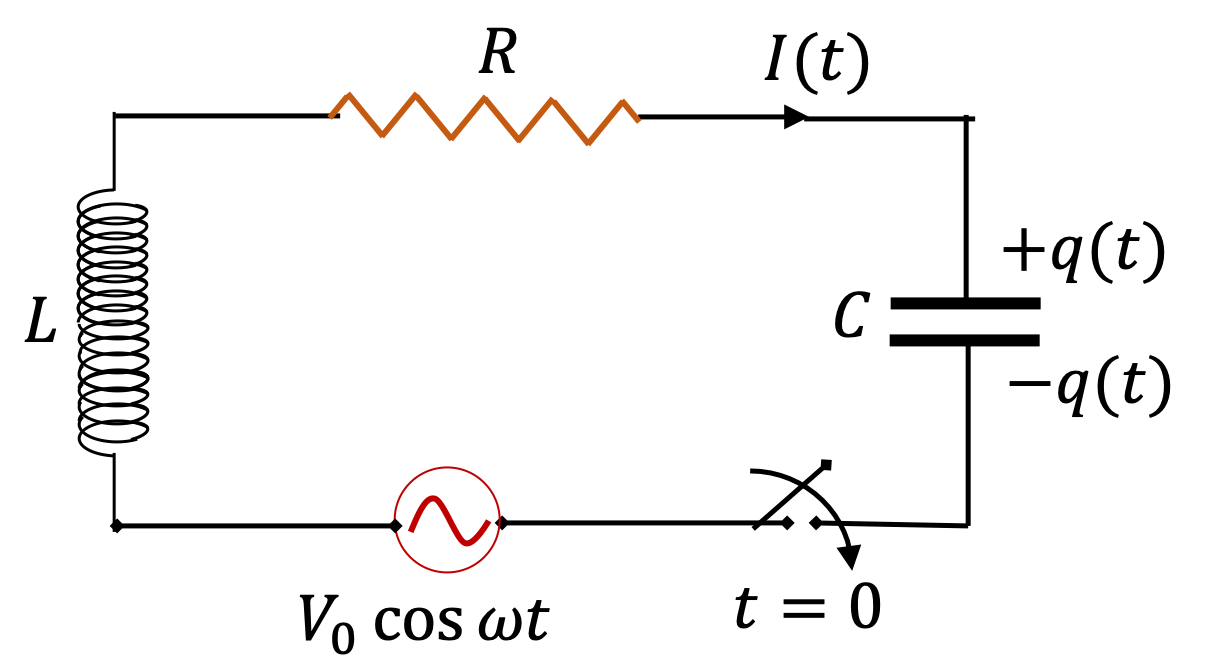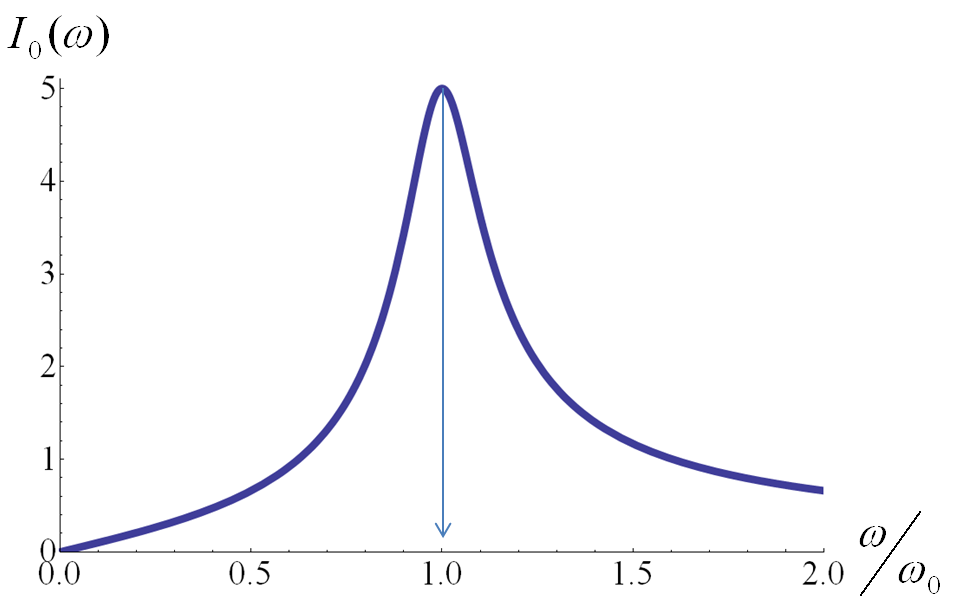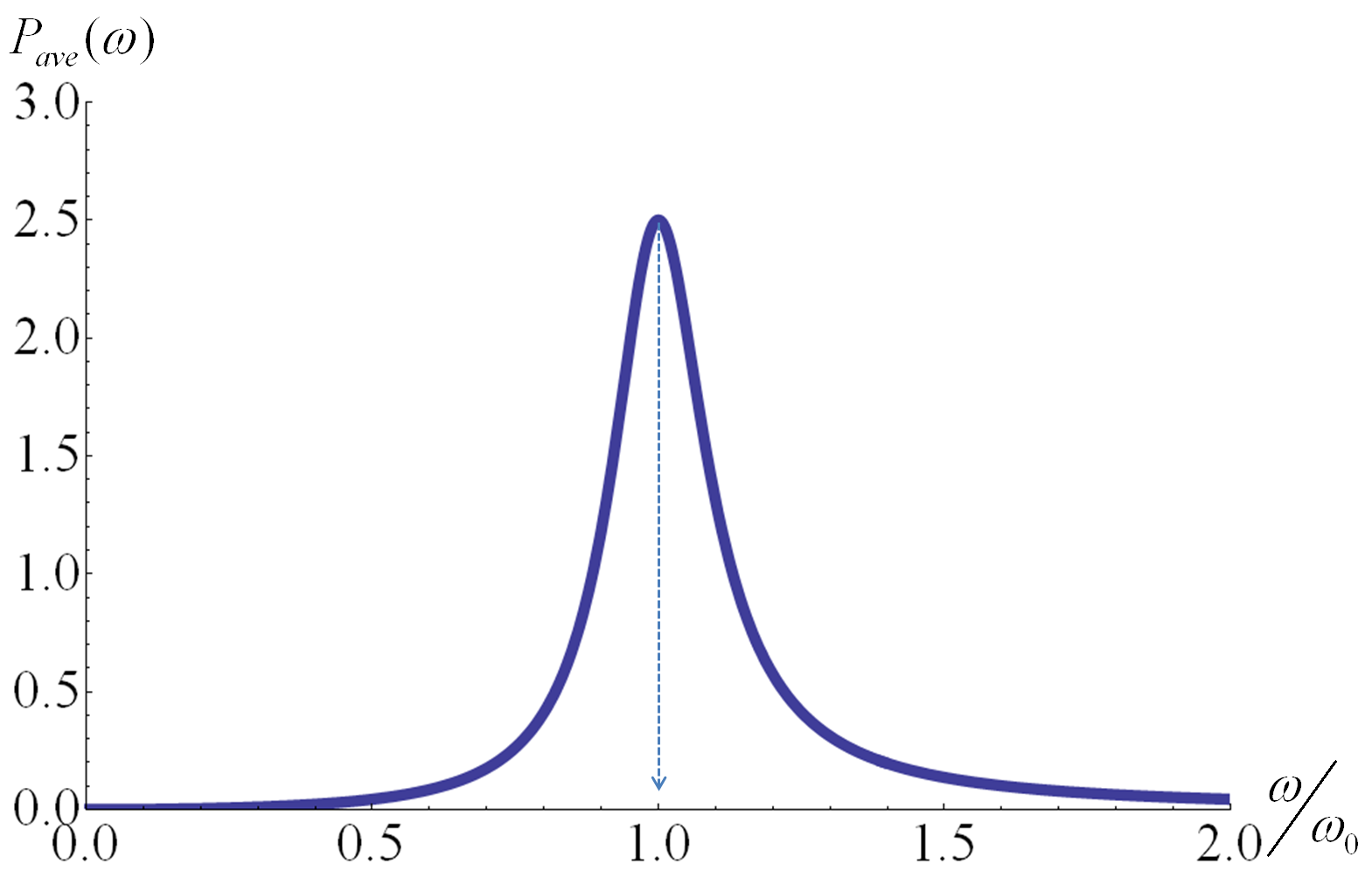## Section43.3Driven Oscillations

The damped oscillator was discussed in Section 43.2, where we saw is that, in a purely damped circuit, with dissipation of energy in the resistor, the charged capacitor becomes uncharged over time. In this section we modify the damped circuit by including an alternating current (AC) generator in the circuit as shown in Figure 43.3.1.Figure 43.3.1. A sinusoidally driven electromagnetic oscillator circuit with resistor $R\text{,}$ inductor $L$ and capacitor $C$ in series with a sinusoidal driving EMF source that varies in time as $V_0 \cos \omega t\text{.}$

The (angular) frequency $\omega$ of the generator is called driving frequency. When you wait “long enough”, the current in the circuit also oscillates at the driving frequency. When that state has reached, we say that the circuit has reached a steady state. For short times after switch is turned on, the circuit behaves in a complicated way. This short-time benavior is called transients.

In practical applications of driven circuits, we work with $R,\ L,\ C$ values corresponding to underdamped circuit. That is,

\begin{equation*} \dfrac{\gamma}{2} \lt \omega_0, \end{equation*}

where, as usual,

\begin{equation*} \gamma = \dfrac{R}{L},\ \ \ \ \omega_0 = \dfrac{1}{ \sqrt{LC} }. \end{equation*}

We will show below that, for $t \gg 2/\gamma\text{,}$ current in the circuit will be

\begin{equation} I(t) = I_0\,\cos(\omega t + \phi_I),\label{driven-circuit-current}\tag{43.3.1} \end{equation}

where

\begin{align} \amp I_0 = \dfrac{V_0}{\sqrt{R^2+\left(\omega L - \dfrac{1}{\omega C} \right)^2}}. \label{driven-circuit-current-amplitude}\tag{43.3.2}\\ \amp \phi_I = \dfrac{\pi}{2} + \tan^{-1} \left(\dfrac{1}{\omega R C} - \dfrac{\omega L}{R} \right). \label{driven-circuit-current-phase}\tag{43.3.3} \end{align}

Here $\phi_I$ is called phase constant of current. The argument of cosine for sinusoidal function is called phase. The phase of the driving EMF in $V_0\cos(\omega t)$ is set to zero as reference. The phase constant $\phi_I$ of current in the circuit is relative to the phase of the driving EMF. Note, to compare phases of two sinusoidal quantities, you need to express both of them either in sine or cosine since every sine can be written as a cosine by

\begin{equation*} \sin(\theta) = \cos(\theta - \pi/2). \end{equation*}

Notice that the denominator on the right side of Eq. (43.3.2) acts as the effective resistance of the series RLC circuit. This effective reistance is called impedance of the circuit. We denote impedance by capital letter $Z\text{.}$

\begin{equation} Z = \sqrt{R^2+\left(\omega L - \dfrac{1}{\omega C} \right)^2}.\label{eq-impedance-of-series-RLC-circuit}\tag{43.3.4} \end{equation}

Current in the circuit varies between $+I_0$ and $-I_0\text{.}$ We call $I_0$ current amplitude. From Eq. (43.3.2), we conclude that current amplitude varies with the driving frequency, $\omega\text{.}$ As you vary $\omega$ of the EMF source, you will change $I_0$ as illustrated in Figure 43.3.2. The driving frequency at which you get the largest amplitude current occurs when

\begin{equation} \omega =\omega_0 = \dfrac{1}{\sqrt{LC}} \equiv \omega_R.\tag{43.3.5} \end{equation}

The observation that a frequency exists at which current fluctuates with the largest amplitude is called resonance of current and the special frequency, $\omega_R\text{,}$ its resonance frequency. When you drive the circuit at the resonance frequency, current makes the largest oscillations about zero. We will see below that at this frequency, source is also able to deliver maximum power into the circuit by examining the resonance of average power.Figure 43.3.2. Resonance of amplitude of current for $R = 0.2\,\Omega\text{,}$ $L= 1\text{ H}\text{,}$ $C = 1\text{ F}\text{,}$ and the peak voltage of the driving EMF set to $V_0 = 1\text{ V}\text{.}$ The peak current in the circuit is maximum when the driving frequency $\omega$ is equal to the natural frequency $\omega_0$ of the circuit.

### Subsection43.3.1(Calculus) Equation of Motion of Driven RLC Circuit

Faraday loop equation for circuit in Figure 43.3.1 gives the following for charge $q(t)$ on the positive plate at an instant when the plate is charging.

\begin{equation} L\dfrac{dI}{dt} + RI + \dfrac{1}{C}\,q = V_0\, \cos \omega t,\label{eq-faraday-loop-equation-driven-circuit}\tag{43.3.6} \end{equation}

with

\begin{equation*} I = \dfrac{dq}{dt}. \end{equation*}

Replacing $I$ in Eq. (43.3.6) we get equation of motion for charge $q(t)\text{.}$

\begin{equation} \dfrac{d^2q}{dt^2} + \gamma\, \dfrac{dq}{dt} + \omega_0^2\,q = \dfrac{V_0}{L}\, \cos \omega t,\label{eq-motion-of-q-driven-circuit}\tag{43.3.7} \end{equation}

where

\begin{equation*} \gamma = \dfrac{R}{L},\ \ \ \ \omega_0 = \dfrac{1}{\sqrt{LC}}. \end{equation*}

For underdamped case, we have $\gamma/2 \lt \omega_0\text{.}$

### Subsection43.3.2(Calculus) Solution of Equation of Motion of Driven RLC Circuit

Eq. (43.3.7) is an inhomogeneoud linear differential equation. Its solution has two parts, which we will denote by $q_c$ and $q_s\text{,}$ with the following exporesions for underdamped case, i.e., when $\gamma/2 \lt \omega_0\text{.}$

\begin{equation} q(t) = q_c(t) + q_s(t),\tag{43.3.8} \end{equation}

with

\begin{align} \amp q_c = e^{-\gamma t / 2} \left( A\, \cos \omega_1 t + B\, \cos \omega_1 t \right),\label{eq-driven-circuit-solution-homogeneous}\tag{43.3.9}\\ \amp q_s = q_\text{amp}\, \cos (\omega t + \phi_q),\label{eq-driven-circuit-solution-steady-state}\tag{43.3.10} \end{align}

where

\begin{align*} \amp q_\text{amp} = \dfrac{V_0/L}{ \sqrt{ (\omega^2 - \omega_0^2)^2 + \gamma^2 \omega^2 } }, \end{align*}

and,

\begin{align*} \tan \phi_q \amp = \dfrac{ \gamma \omega }{\omega^2 - \omega_0^2 }, \\ \amp = \dfrac{ 1 }{(\omega L/R) - (1/\omega RC) }, \end{align*}

and $A$ and $B$ are constants to be fixed using initial values of charge $q$ and current $I\text{.}$ Current $I$ at arbitrary time is obtained by

\begin{equation*} I = \dfrac{dq}{dt}. \end{equation*}

In Eq. (43.3.9), you can see that, with time, the multiplicative negative exponetial will make it negligible after $t \gt \gt 2/\gamma\text{.}$

\begin{equation*} q_c(t)\rightarrow 0 \text{ as } t\gt \gt 2/\gamma. \end{equation*}

This leaves $q_s$ part the only part, which is the steady state solution. We notice that $q_s(t)$ is sinusoidal with same frequency as the driving EMF.

\begin{equation*} q(t) \rightarrow q_s(t) \text{ as } t\gt \gt 2/\gamma. \end{equation*}

By taking time derivative of $q_s$ we get steady state current, $I_s\text{.}$

\begin{align*} I_s \amp = \dfrac{dq_s}{dt} = -\omega q_\text{amp}\, \sin (\omega t + \phi_q), \\ \amp = \omega q_\text{amp}\, \cos (\omega t + \phi_q + \pi/2), \\ \amp = \omega I_0\, \cos (\omega t + \phi_I), \end{align*}

where current amplitude,

\begin{align*} I_0 \amp = \omega q_\text{amp} \\ \amp = \omega \dfrac{V_0/L}{ \sqrt{ (\omega^2 - \omega_0^2)^2 + \gamma^2 \omega^2 } } \\ \amp = \dfrac{V_0}{ \sqrt{ R^2 + \left(\omega L - \dfrac{1}{\omega C}\right)^2 } }, \end{align*}

and the phase constant,

\begin{align*} \tan \phi_I \amp = \tan (\phi_q + \pi/2 )\\ \amp = -1.0/\tan \phi_q = \left( \dfrac{1}{\omega R C} - \dfrac{\omega L}{R}\right) . \end{align*}

### Subsection43.3.3Power Delivered to the Circuit by the EMF Source

The practical importance of the resonance phenomenon is seen dramatically in the choice of the frequency of the driving power source in delivering power to the rest of the circuit. The energy at any instant is stored in the capacitor and the inductor, but only the resistor in the circuit dissipates energy. Therefore, the average energy used by the circuit during an interval will be equal to the product of the instantaneous power into the resistor and the interval of time. Thus, the energy deposited in the resistor in an interval of time between $t$ to $t+dt$ will be given by

\begin{equation*} P(t) dt = I(t) V_R(t), \end{equation*}

where $I(t)$ is the current through the resistor and $V_R$ is the voltage drop across the resistor. From Ohm's law we can replace $V_R$ by $I R$ and obtain

\begin{equation*} P(t) dt = I_0^2 R\cos^2(\omega t+\phi_I), \end{equation*}

where $\phi_I$ is the phase of the current relative to the phase of the driving EMF. The energy deposited in the resistor per cycle is obtained by integrating this expression over a period, $T = 2\pi/\omega\text{.}$

\begin{equation*} \text{Energy(one cyle)}=\int_0^{T}P(t) dt = \dfrac{1}{2}I_0^2 RT. \end{equation*}

Therefore, the average rate at which the energy is dissipated in the resistor will be $\dfrac{1}{2}I_0R^2\text{.}$ This is the average power dissipated.

\begin{equation*} P_{\text{ave}} = \dfrac{\text{Energy(one cyle)}}{T} = \dfrac{1}{2}I_0^2R. \end{equation*}

Since the peak current $I_0$ is a function of the driving frequency $\omega\text{,}$ the average power delivered would also be a function of the frequency $\omega$ of the EMF source. Thus, the average power is maximum at the same frequency at which the peak current is maximum. We saw above that the peak current was maximum when the source EMF was oscillating at the same frequency as the natural frequency of the circuit; see Figure 43.3.3.Figure 43.3.3. The resonance curve of the average power dissipated in the resistor for $R$ = 0.2 $\Omega\text{,}$ $L$= 1 H, $C$ = 1 F, and the peak voltage of the driving EMF set to $V_0 = 1$ V. The peak power is delivered when the driving frequency $\omega$ is equal to the natural frequency $\omega_0$ of the circuit.

The curve shows that the maximum power is delivered to the circuit when the circuit is driven at the natural frequency. Denoting the resonance frequency of power as $\omega_R$ we have

\begin{equation*} \omega_R = \omega_0 = \dfrac{1}{\sqrt{LC}}\ \ \ \text{(Resonance of Power.)} \end{equation*}

This formula for the average power in the resistor differs from the power for the constant current circuit we had found in an earlier chapter. There, we had found that the power dissipated in a resistor is $I^2R\text{,}$ but here we find that the average power is $\dfrac{1}{2}I_0^2R$ where $I_0$ is the amplitude of the oscillating current, which changes between $-I_0$ and $+I_0$ sinusoidally in time. It is possible to define an averaged current, called the root-mean squared current, or the RMS-current, we can rewrite the average power $P_{\text{ave}}$ as a formula that is similar to a DC circuit.

\begin{equation*} I_{\text{rms}} = \dfrac{I_0}{\sqrt{2}} \end{equation*}

This will give the average power dissipated as

\begin{equation*} P_{\text{ave}} = R\: I_{\text{rms}}^2. \end{equation*}

Find frequencies at which current in each circuit of Figure 43.3.5 will resonate.

Hint

$f_R = \omega_R/2\pi\text{,}$ $\omega_R=1/\sqrt{LC}\text{.}$

(a) $796\:\text{Hz}\text{,}$ (b) $1125\:\text{Hz}$ (c) $1989\:\text{Hz}\text{,}$ (d) $1592\:\text{Hz}\text{.}$

Solution

(a)

\begin{equation*} \omega_R = \dfrac{1}{\sqrt{LC}} = \dfrac{1}{\sqrt{10\times 10^{-3}\:\text{H}\times 4\times 10^{-6}\text{F}}} = 5000\:\text{sec}^{-1}. \end{equation*}

This give $f_R = \omega_R/2\pi = 796\:\text{Hz}\text{.}$

(b)

\begin{equation*} \omega_R = \dfrac{1}{\sqrt{LC}} = \dfrac{1}{\sqrt{1\times 10^{-3}\:\text{H}\times 20\times 10^{-6}\text{F}}} = 7071\:\text{sec}^{-1}. \end{equation*}

This give $f_R = \omega_R/2\pi = 1125\:\text{Hz}\text{.}$

(c)

\begin{equation*} \omega_R = \dfrac{1}{\sqrt{LC}} = \dfrac{1}{\sqrt{4\times 10^{-3}\:\text{H}\times 1.6\times 10^{-6}\text{F}}} = 12500\:\text{sec}^{-1}. \end{equation*}

This give $f_R = \omega_R/2\pi = 1989\:\text{Hz}\text{.}$

(d)

\begin{equation*} \omega_R = \dfrac{1}{\sqrt{LC}} = \dfrac{1}{\sqrt{5\times 10^{-3}\:\text{H}\times 2\times 10^{-6}\text{F}}} = 10000\:\text{sec}^{-1}. \end{equation*}

This give $f_R = \omega_R/2\pi = 1592\:\text{Hz}\text{.}$

For each circuit in Checkpoint 43.3.4, find the amplitude of the current if the peak voltage of the source of frequency $600\text{ Hz}$ is $10\text{ V}\text{.}$

Hint

Use $I_0 = \dfrac{V_0}{Z}$

(a) $20\text{ mA}\text{,}$ (b) $730\text{ mA}\text{,}$ (c) $66\text{ mA}\text{,}$ and (d) $31\text{ mA}\text{.}$

Solution

We use the result for a series $RLC$ circuit.

\begin{equation*} I_0 = \dfrac{V_0}{Z},\ \ \ \ Z = \sqrt{R^2 + (\omega L - 1/\omega C)^2}. \end{equation*}

where $\omega = 2 \pi f$ with $f$ the frequency of the source EMF. Putting the numerical values from each figure we find the following values for the peak current in the four cases: (a) $20\text{ mA}\text{,}$ (b) $730\text{ mA}\text{,}$ (c) $66\text{ mA}\text{,}$ and (d) $31\text{ mA}\text{.}$

For each circuit in Checkpoint 43.3.4, find the average power delivered to the circuit if the peak voltage of the source of frequency $600\text{ Hz}$ is $10\text{ V}\text{.}$

Hint

$P_{\textrm{ave}} = \frac{1}{2}RI_0^2 \text{.}$

(a) $0.8\text{ W}\text{,}$ (b) $2.7\text{ W}\text{,}$ (c) $0.011\text{ W}\text{,}$ and (d) $0.14\text{ W}\text{.}$

Solution

Average power is related to the peak current and resistance only: $P_{\textrm{ave}} = \frac{1}{2}RI_0^2\text{,}$ where $I_0$ is the peak current. The peak current for each circuit has been worked out in the last exercise. Now, using this formula we obtain the following values for the average power: (a) $0.8\text{ W}\text{,}$ (b) $2.7\text{ W}\text{,}$ (c) $0.011\text{ W}\text{,}$ and (d) $0.14\text{ W}\text{.}$

Consider the circuit given in Figure 43.3.9.

(a) Find the steady state current in the circuit.

(b) Find the resonance frequency of current.

(c) Find the voltage across the resistor in the steady state.

(d) (Calculus) Find the voltage drop across the capacitor in the steady state.

(e) (Calculus) Find the back emf in the inductor in the steady state.

Hint

Use formulas presented in this section.

(a) $I(t) = (7.2\text{ mA})\, \cos\left( 200\,t + 1.57\right) \text{,}$ (b) $\omega_R = \omega_0 = 1921\text{ sec}^{-1}\text{,}$ or $306\text{ Hz}\text{.}$ (c) $(36\text{ mV})\, \cos\left( 200\,t + 1.57\right)\text{,}$ (d) $12\text{ V} \,\sin(200\,t + 1.57)\text{,}$ (e) $-5.76\text{ mV} \sin(200\, t + 1.57) \text{,}$

Solution 1 (a)

Let us compute the constants we will need.

\begin{align*} \amp \gamma = \dfrac{R}{L} = 1250\text{ sec}^{-1}. \\ \amp \omega_0 = \dfrac{1}{\sqrt{RC}} = 1921\text{ sec}^{-1}. \\ \amp Z = \sqrt{R^2 + ( \omega L - 1/(\omega C) )^2 } = 1669\ \Omega. \end{align*}

The current amplitude

\begin{equation*} I_0 = \dfrac{V_0}{Z} = \dfrac{12\text{ V}}{1669\ \Omega} = 7.2\text{ mA}. \end{equation*}

The phase constant for current

\begin{align*} \phi_I \amp = \tan^{-1}\left( \dfrac{1}{\omega R C} - \dfrac{\omega L}{R } \right)\\ \amp = 1.57\text{ rad}. \end{align*}

Therefore, steady starte current at instant $t$ will be

\begin{equation*} I(t) = (7.2\text{ mA})\, \cos\left( 200\,t + 1.57\right). \end{equation*}
Solution 2 (b)

(b) Resonance angular frequency of current will be

\begin{equation*} \omega_R = \omega_0 = 1921\text{ sec}^{-1}. \end{equation*}

This would give the regular frequency

\begin{equation*} f_R = \dfrac{\omega_R}{2\pi} = 306\text{ Hz}. \end{equation*}
Solution 3 (c)

(c) Voltage across resistor is simply $IR\text{.}$

\begin{equation*} V _R = (36\text{ mV})\, \cos\left( 200\,t + 1.57\right). \end{equation*}
Solution 4 (d)

(d) Voltage across capacitor is a little tricky since $V_C = q/C$ with $q = \int I(t) dt\text{.}$

\begin{align*} V_C \amp = \dfrac{1}{C}\int I(t) dt\\ \amp = \dfrac{1}{C}\int I_0\,\cos(\omega t + \phi_I) dt\\ \amp = \dfrac{I_0}{\omega C} \,\sin(\omega t + \phi_I) \\ \amp = 12.006\text{ V} \,\sin(200\,t + 1.57) \end{align*}
Solution 5 (e)

(e) Back EMF by the inductor is also a little tricky since $\mathcal{E}_\text{back} = L dI/dt\text{.}$

\begin{align*} \mathcal{E}_\text{back} \amp = L \dfrac{dI}{dt}\\ \amp = L I_0\, \dfrac{d \cos(\omega t + \phi_I)}{dt}\\ \amp = -\omega L I_0 \sin(\omega t + \phi_I) \\ \amp = -5.76\text{ mV} \sin(200\, t + 1.57) \end{align*}